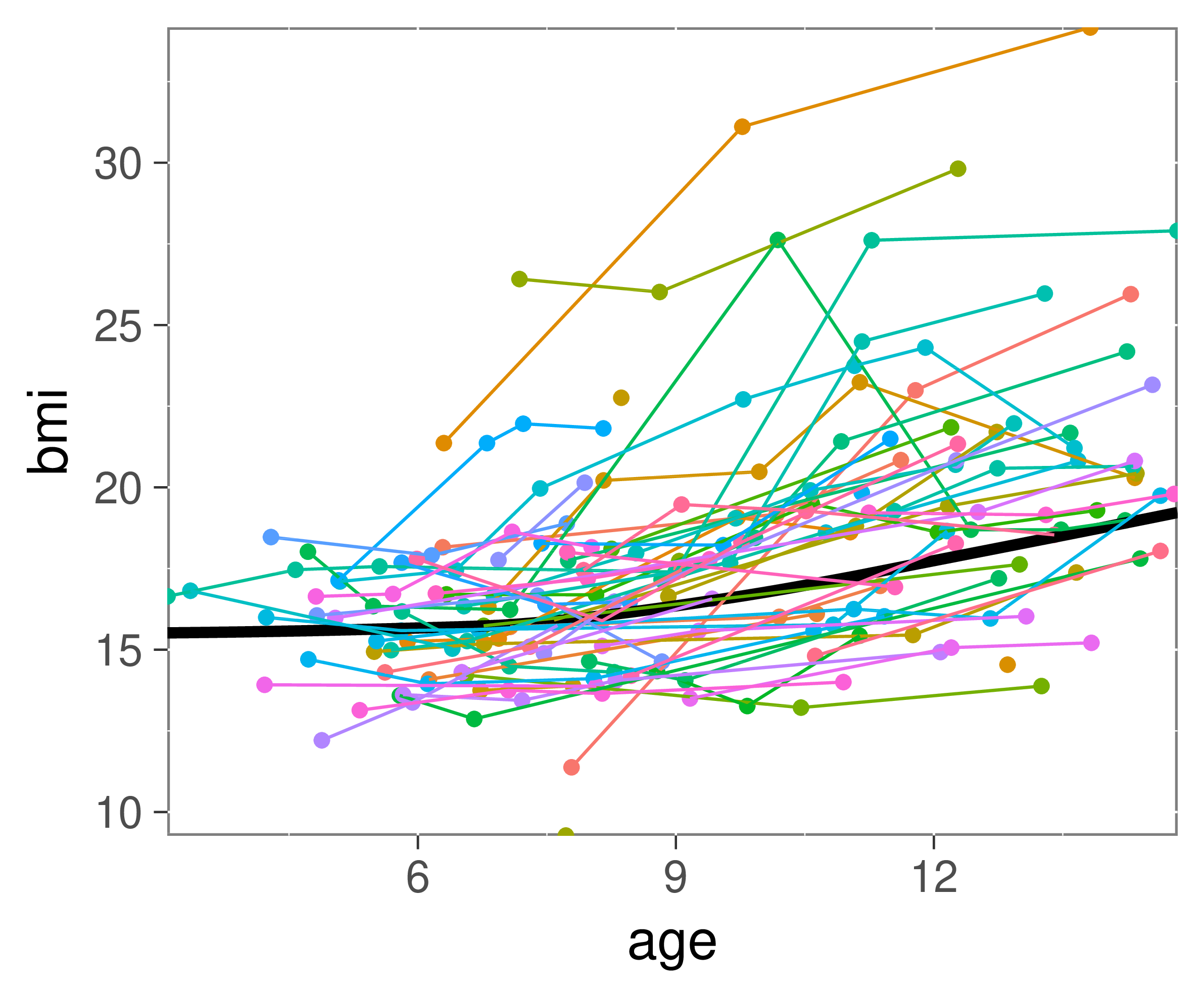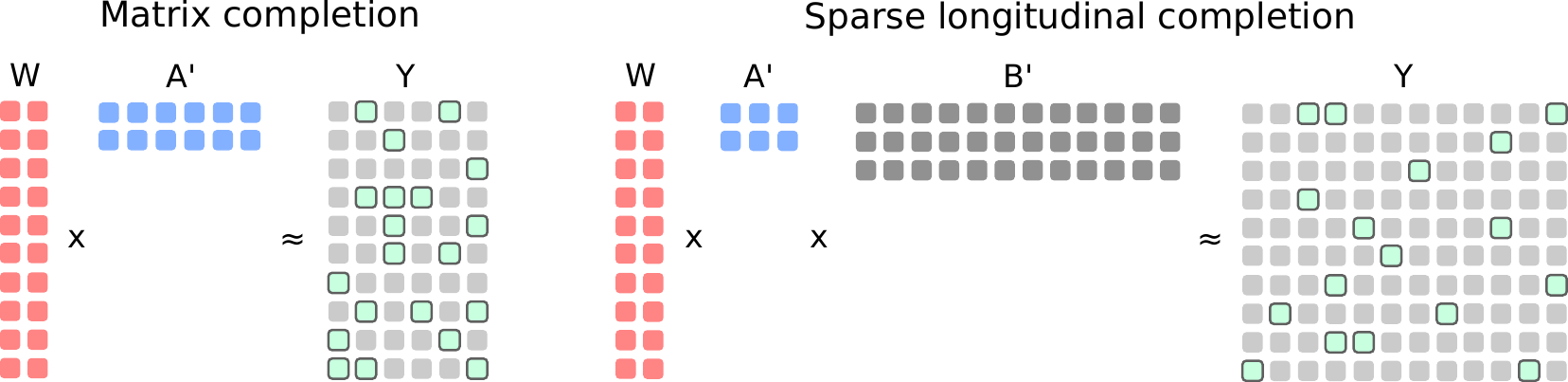# fcomplete

Suppose we observe N subjects, each subject at multiple timepoints and we want to estimate a trajectory of progression of measurements in individual subjects. For example, suppose you observe BMI of N children at different ages, as presented belowHere, the connected dots come from individual subjects and the black thick line corresponds to the population mean.

I’ve build the package to fit trajectories using matrix completion and I described the methodology in my recent paper Kidziński, Hastie (2018). To this end, we discretize the time grid some continous basis and find a low-rank decomposition of the dense matrix.In the classical matrix completion, we look for matrices W and A that fit the observed points in Y (green points in the image above). In our method, in order to impose smoothness, we additionaly assume the basis B and again we look for the reprezentation minimizing the errror.

The interface of the package is based on the mixed-effect models in R. In particular, if we are given temporal observations Y in the long format with columns id, age and bmi, while additional covariates X, constant over time are given as a data frame with columns id and, say, gender, we can fit the model by writing

model = fregression(bmi ~ age + gender | id, data = Y, covariates = X)
print(model)


For more information, please refer to the manual and to vignettes.

## Publications

. Longitudinal data analysis using matrix completion. Under review, 2018.